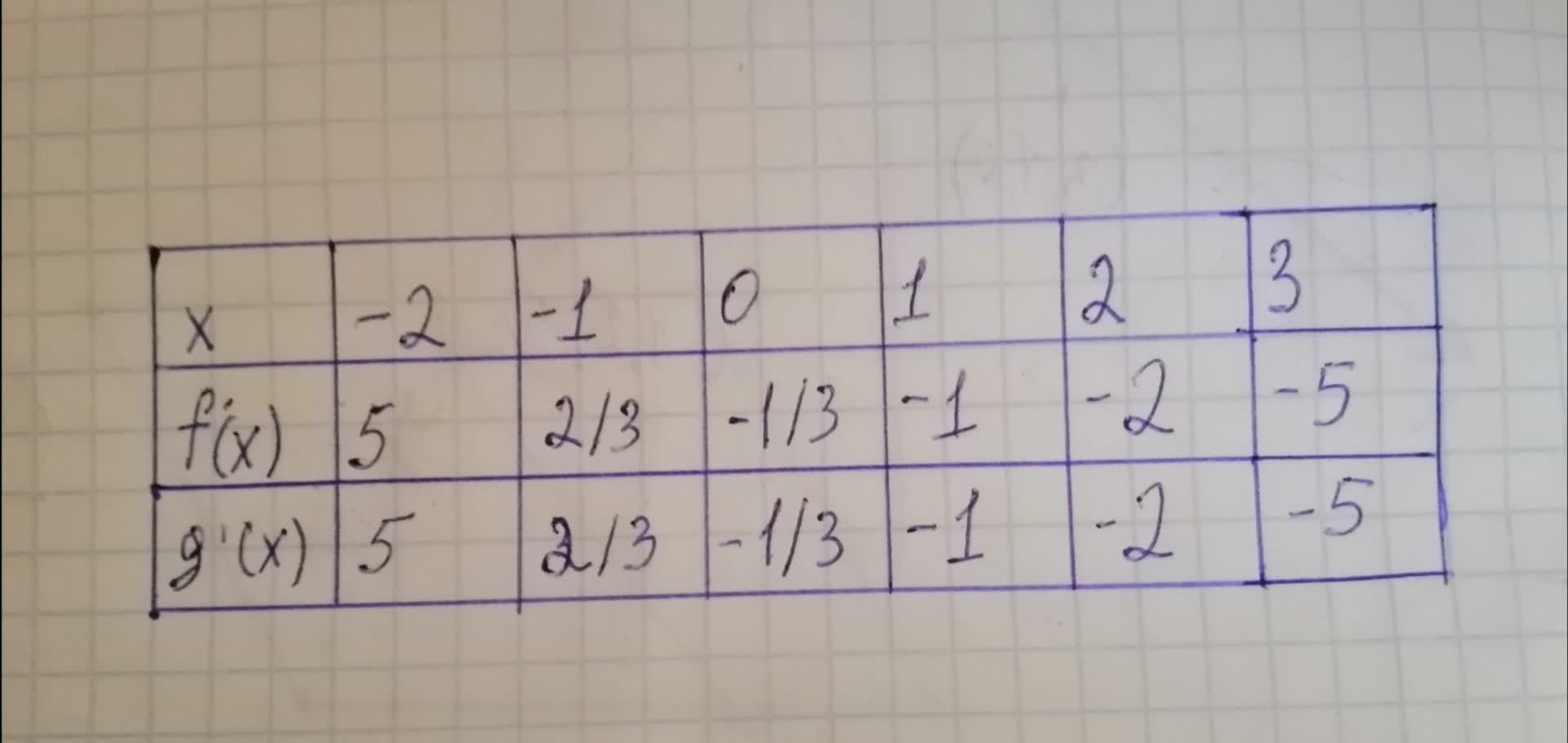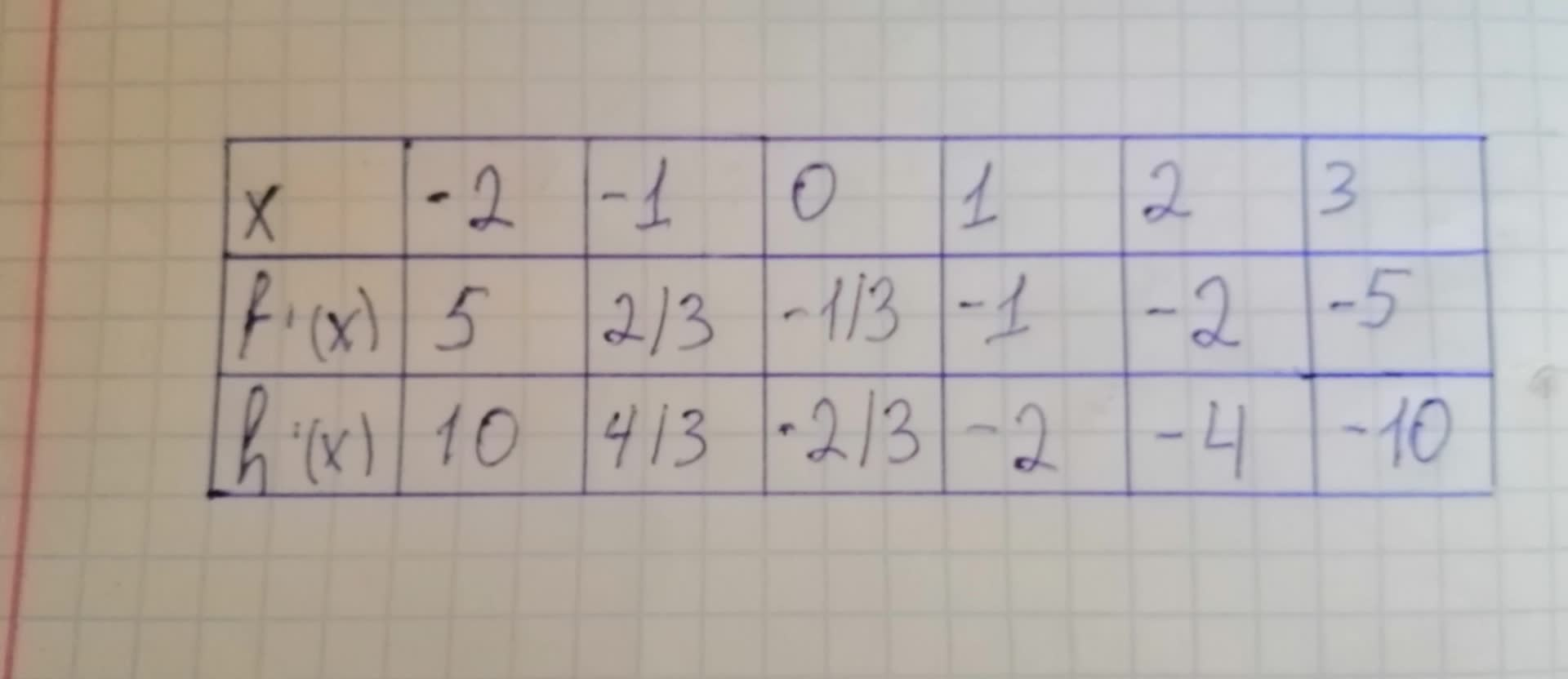# The table shows some values of the derivative of an unknown function f.Complete the table by finding the derivative of each transformation of f, it possible a) g(x) = f(x) - 2 b) h(x) = 2 f(x) c) r(x) = f(-3x)abondantQ 2021-01-22 Answered
The table shows some values of the derivative of an unknown function f.Complete the table by finding the derivative of each transformation of f, it possible
a)
b) $h\left(x\right)=2f\left(x\right)$
c) $r\left(x\right)=f\left(-3x\right)$
You can still ask an expert for help

• Questions are typically answered in as fast as 30 minutes

Solve your problem for the price of one coffee

• Math expert for every subject
• Pay only if we can solve itottcomn
Step 1
The derivative properties:

$\frac{d}{dx}\left(af\left(x\right)\right)=a{f}^{\prime }\left(x\right)$
calculate the derivative of with respect to x as follows

$={f}^{\prime }\left(x\right)$Step 2
Now calculate the derivative of $h\left(x\right)=2f\left(x\right)$ with respect to x as follows
${h}^{\prime }\left(x\right)=\frac{d}{dx}=\left(2f\left(x\right)\right)$
$=2{f}^{\prime }\left(x\right)$
$\therefore {h}^{\prime }\left(x\right)=2{f}^{\prime }\left(x\right)$Step 3
Now calculate the derivative of $r\left(x\right)=f\left(-3x\right)$ with respect to x as follows
${r}^{\prime }\left(x\right)=\frac{d}{dx}=f\left(-3x\right)$

As compute the the value of
$-3{f}^{\prime }\left(-3x\right)$

$=-3{f}^{\prime }\left(6\right)$
Here cannot be computed the values
of ${f}^{\prime }\left(6\right)$ is not known.

$=15$

$=-3\left(-\frac{1}{3}\right)$
$=1$
And

Hense $-3{f}^{\prime }\left(-3\right)$ can not be compluted.